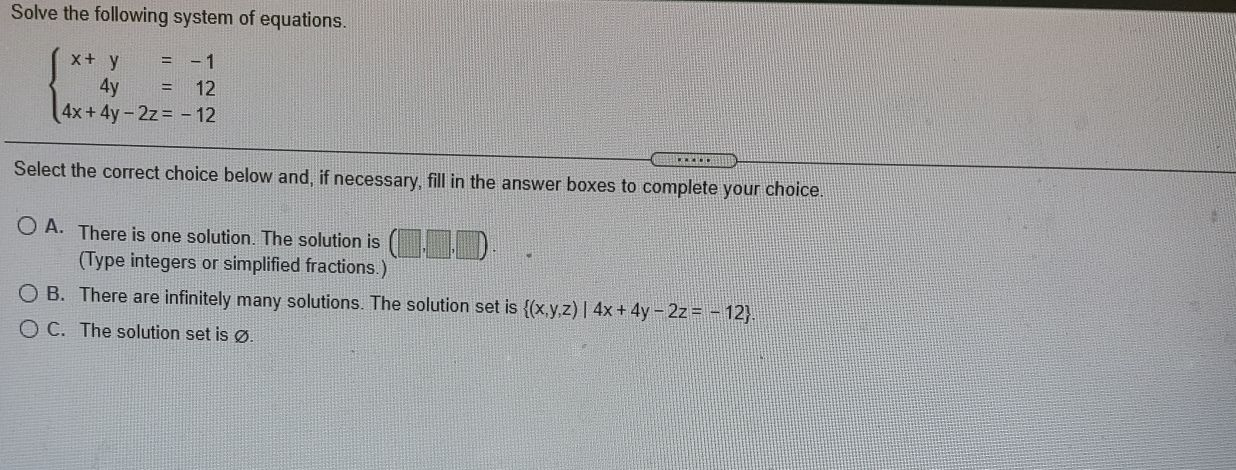### Still have math questions?

Algebra
QuestionSolve the following system of equations.

$$\left\{ \begin{array} { r r } { x + y } & { = - 1 } \\ { 4 y } & { = 12 } \\ { 4 x + 4 y - 2 z } & { = - 12 } \end{array} \right.$$

Select the correct choice below and, if necessary, fill in the answer boxes to complete your choice.

A. There is one solution. The solution is (_ , _ , _) .

B. There integers or simplified fractions.)

C. The solution set is $$\varnothing$$ .